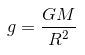# The relation between g and G is______________

The G (Universal Gravitational Constant) and g (Acceleration due to gravity) are distinct entities in physics.

## Acceleration due to gravity(g)

The acceleration experienced by a body under free fall is due to the gravitational force of the massive body.

• It changes from place to place.
• Acceleration due to the gravity of the earth is 9.8 m/s2

## Universal Gravitational Constant(G)

The force of attraction between two objects with unit mass separated by a unit distance at any part of this universe.

• It is constant at any point in this universe.
• G = 6.67×10-11 Nm2/kg

## Relationship Between G and g

The relationship between G (universal gravitational constant) and g (acceleration due to gravity)

is that:Where,

• g is the acceleration due to the gravity of any given body in m.s-2
• G is the universal gravitational constant in Nm2.kg-2
• R is the radius of the given body in km
• M is the mass of the given body in kg

### Articles to Explore:(26)(1)